# Intersection homology

For non-singular complex projective algebraic varieties there are a number of (co)homological properties, such as Poincaré duality, Hodge decomposition, hard Lefschetz theoremthat are no longer true for the ordinary (co)homology of singular varieties. Intersection (co)homology is a modification of the usual theory designed to retain such properties for the case of singular varieties with, initially, special stress on Poincaré duality in its homological (intersection) form: Letbe a compact oriented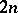-dimensional manifold, and let,be homology classes with representative cyclesandthat intersect in finitely many points (such representation cycles exist). Then the number of points of intersection counted with their multiplicities is independent ofand, and this defines a duality pairing.

Letbe an-dimensional complex-analytic variety (possibly with singularities, cf. also Analytic manifold) with a Whitney stratification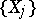. Letbe the codimension of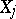in. Let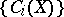be one of the usual complexes of geometric chains on(for instance,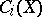could be the piecewise-linear-chains with respect to some piecewise-linear structure on). The complex of intersection chains is defined as the subcomplexsatisfying the condition: a chain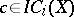meets each singular stratumin a set of real dimensionand its boundary intersects each singular stratumin a set of real dimension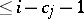.

The-th intersection homology group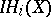is the-th homology group of the chain complex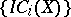, [a1].

There is also a sheaf-theoretic approach to intersection (co)homology. This involves perverse sheafs [a4], [a5], [a9] (cf. also-module).

There are many applications of intersection (co)homology, in particular to representation theory [a1], [a5], [a8] (for instance, a proof of the Kazhdan–Lusztig conjecture, [a7]).

Another beautiful and very useful property of smooth closed oriented Riemannian (or triangulated) manifolds is that the-th real cohomology group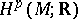is isomorphic to the zero eigen space of the appropriate Laplace operator (harmonic cochains). To have something similar for open manifolds, an appropriate "functional cohomology" theory has to be developed. This led to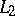-cohomology.

Letbe any (in general, incomplete) Riemannian manifold with metricand without boundary. Letbe the space of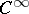-forms onand let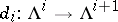be exterior differentiation. Letbe the space of square-integrable-forms with measurable coefficients. The-cochain complex is now defined by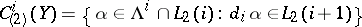, and (one definition of) the-th-cohomology group of,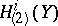, is as follows:is the-th cohomology group of this cochain complex [a2]. In general these cohomology groups depend on the metric.

Letbe again a complex-analytic variety and let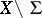be its non-singular part. In many cases the-cohomology of(with respect to an appropriate metric) has been found to be the dual of the intersection homology of, [a1][a3], [a10], [a11].